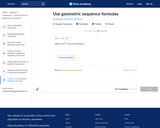Updating search results...

# 19 Results

View
Selected filters:
• NC.M1.F-IF.3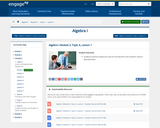Conditional Remix & Share Permitted
CC BY-NC-SA
Rating
0.0 stars

Students examine sequences and are introduced to the notation used to describe them.

Subject:
Math 1
Mathematics
Material Type:
Lesson
Author:
EngageNY
02/03/2020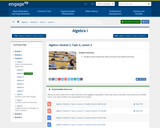Conditional Remix & Share Permitted
CC BY-NC-SA
Rating
0.0 stars

Students write sequences with recursive and explicit formulas.

Subject:
Math 1
Mathematics
Material Type:
Lesson
Author:
EngageNY
02/09/2020Rating
0.0 stars

Lesson plans and activities which address the concept of arithmetic sequences, finishes arithmetic sequences and applies the idea of arithmetic sequences.

Subject:
Mathematics
Material Type:
Unit of Study
Provider:
Project Maths
02/26/2018Conditional Remix & Share Permitted
CC BY-NC-SA
Rating
0.0 stars

Solve a series of arithmetic sequences in this Millionaire Maker game.&nbsp;&nbsp;

Subject:
Math 1
Material Type:
Activity/Lab
Author:
KORI ELLIOTT
06/01/2020Conditional Remix & Share Permitted
CC BY-NC-SA
Rating
0.0 stars

Solve a series of arithmetic sequences in this Millionaire Maker game.&nbsp;&nbsp;

Subject:
Math 1
Material Type:
Activity/Lab
Assessment
Formative Assessment
Interactive
Author:
Christopher Smith
04/09/2020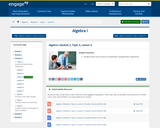Rating
0.0 stars

Students learn the structure of arithmetic and geometric sequences.

Subject:
Mathematics
Material Type:
Lesson Plan
Provider:
EngageNY
04/03/2018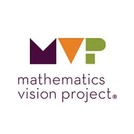Unrestricted Use
CC BY
Rating
0.0 stars

This task builds upon students’ experiences with arithmetic and geometric sequences to
extend to the broader class of linear and exponential functions with continuous domains. The term
“domain” should be introduced and used throughout the whole group discussion. Students are
given contextual situations that can be modeled with either discrete and continuous linear
functions, or discrete and continuous exponential functions. They are also asked to compare these
types of functions using various representations.

Subject:
Math 1
Mathematics
Material Type:
Activity/Lab
Author:
The Mathematics Vision Project
02/23/2020Rating
0.0 stars

In this Khan Academy activity, students will find the next term in an arithmetic sequence.

Subject:
Mathematics
Material Type:
Activity/Lab
Provider:
08/27/2018Rating
0.0 stars

In this Khan Academy activity, students will find the next term in a geometric sequence.

Subject:
Mathematics
Material Type:
Activity/Lab
Provider:
08/27/2018Rating
0.0 stars

In this Khan Academy activity, students will find the next term in a geometric sequence.

Subject:
Mathematics
Material Type:
Activity/Lab
Provider:
08/27/2018Rating
0.0 stars

Students will compare patterns of arithmetic and geometric sequences, and then use the geometric sequence formula to solve practice problems.

Subject:
Mathematics
Material Type:
Activity/Lab
Provider:
study.com
11/27/2017Only Sharing Permitted
CC BY-NC-ND
Rating
0.0 stars

Students will use a video about two rival competitive eaters to explore linear and quadratic patterns.

Subject:
Algebra
Math 1
Mathematics
Material Type:
Activity/Lab
Formative Assessment
Author:
Robert Kaplinsky
11/26/2019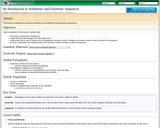Rating
0.0 stars

This lesson is designed to introduce students to the arithmetic and geometric sequences.

Subject:
Mathematics
Material Type:
Activity/Lab
Provider:
Shodor Education Foundation
02/27/2018Conditional Remix & Share Permitted
CC BY-NC-SA
Rating
0.0 stars

This task provides opportunities for students to show their understanding of functions in various representations by making matches (3 cards in a set).

Subject:
Math 1
Mathematics
Material Type:
Formative Assessment
Author:
Mathematics Vision Project
03/10/2020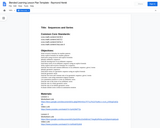Unrestricted Use
CC BY
Rating
0.0 stars

This is an Algebra 2 Unit that explores sequences and series.  This includes arithmetic, geometric, and other sequences, as well as recursive and explicit formulas that generate these sequences.  Arithmetic, finite geometric, and infinite geometric series are also covered in this unit.  This unit is designed as a flipped instructional unit.  Worksheets and video links are provided.

Subject:
Algebra
Mathematics
Material Type:
Unit of Study
Provider:
Michigan Virtual
Author:
Raymond Herek
07/29/2016Unrestricted Use
CC BY
Rating
0.0 stars

The purpose of this task is for students to explicitly consider when a discrete or continuous model
is appropriate for a given context. The situations in this task are designed to contrast discrete and
continuous models for both linear and exponential functions. The task also provides opportunity
for students to model with mathematics by connecting the type of change, either linear or
exponential with the nature of that change, either discrete or continuous.

Subject:
Math 1
Mathematics
Material Type:
Activity/Lab
Author:
The Mathematics Vision Project
02/23/2020Conditional Remix & Share Permitted
CC BY-NC-SA
Rating
0.0 stars

This task is designed for students to practice their understanding of function. After reviewing various naming conventions of function (“versus”, “with respect to”, “over”, “dependent on”), students are to determine whether or not each situation is a function, then justify their answer.

Subject:
Math 1
Mathematics
Material Type:
Lesson
Author:
Mathematics Vision Project
03/10/2020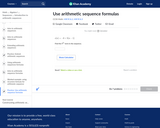Rating
0.0 stars

In this Khan Academy activity, students will find the nth term in an arithmetic sequence.

Subject:
Mathematics
Material Type:
Activity/Lab
Provider:
08/27/2018